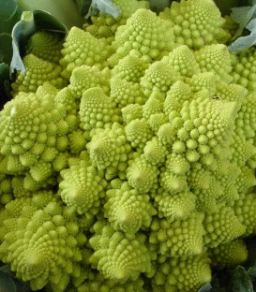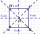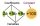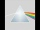# Fraction unknowns

Divide of fractions with unknowns:

Fraction 1: The quantity x squared plus 6 times x plus 9 over the quantity x minus 1.
Fraction 2: the quantity x squared minus 9 over the quantity x squared minus 2 times x plus 1.

Find Fraction 1 over Fraction 2.

d =  0

### Step-by-step explanation:Did you find an error or inaccuracy? Feel free to write us. Thank you!Tips to related online calculators
Need help to calculate sum, simplify or multiply fractions? Try our fraction calculator.

## Related math problems and questions:

• The quotient 2The quotient of the quantity k minus 10 and m
• A fractionI think of a fraction, if we increase its numerator and denominator by one, the value of the fraction increases by one tenth, what fraction do I think?
• Fraction expressionWhich expression is equivalent to : minus 9 minus left parenthesis minus 4 start fraction 1 divided by 3 end fraction right parenthesis
• SelectSelect the statement that describes this expression: 5 x (3 + 4) - 4. a) 5 times the sum of 3 and 4, then subtract 4 b) 5 times 3 plus 4 minus 4 c) 5 more than the sum of 3 and 4, and then subtract 4 d) 5 times 3 plus the difference of 3 and 4 What is the
• Extending square gardenMrs. Petrová's garden had the shape of a square with a side length of 15 m. After its enlargement by 64 m2 (square), it had the shape of a square again. How many meters has the length of each side of the garden been extended?
• Flowerbed 2Around the square flower bed in a park is sidewalk 2 m wide. The area of ​​this sidewalk is 243 m2. What is the area of the flowerbed?
• Expressions with variableThis is algebra. Let n represent an unknown number and write the following expressions: 1. 4 times the sum of 7 and the number x 2. 4 times 7 plus the number x 3. 7 less than the product of 4 and the number x 4. 7 times the quantity 4 more than the number
• Equation with fractionsWhat is the simplified form of the equation fraction 4 over 5 n minus fraction 1 over 5 equals fraction 2 over 5 n? n = -2 n = 4 n = fraction 1 over 2
• The cubeThe cube has a surface area of 216 dm2. Calculate: a) the content of one wall, b) edge length, c) cube volume.
• Surface area of the topA cylinder is three times as high as it is wide. The length of the cylinder’s diagonal is 20 cm. Find the surface area of the top of the cylinder.
• What is 10What is the measure of the square side whose area is (4x2 + 28x+49) square units?
• Simplest formFind the simplest form of the following expression: 3 to the 2nd power - 1/4 to the 2nd power.Please determine the solvability conditions of the equation, solve the equation and perform the test: x divided by x squared minus 2x plus1 the whole minus x + 3 divided by x squared minus 1 this is equal to 0: x/(x2-2x+1) - (x+3)/( x2-1) = 0Evaluate following expression: 5 to the power of 2 - (4x4) divided by 2 =What is the sign of expression: minus 18 start fraction 9 divided by 17 end fraction plus left parenthesis minus 18 start fraction 9 divided by 17 end fraction right parenthesis?Find the number x, which if it increases by 2, then its square increases by 21 percent.Find the surface of the cube with a volume of 27 dm3.